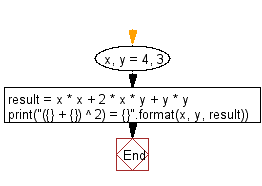﻿ Python: Python program to solve (x + y) * (x + y) - w3resource# Python: Python program to solve (x + y) * (x + y)

## Python Basic: Exercise-38 with Solution

Write a Python program to solve (x + y) * (x + y).

Test Data : x = 4, y = 3
Expected Output : (4 + 3) ^ 2) = 49

Sample Solution:

Python Code:

``````x, y = 4, 3
result = x * x + 2 * x * y + y * y
print("({} + {}) ^ 2) = {}".format(x, y, result))
```
```

Sample Output:

```(4 + 3) ^ 2) = 49
```

Flowchart:## Visualize Python code execution:

The following tool visualize what the computer is doing step-by-step as it executes the said program:

Python Code Editor:

Have another way to solve this solution? Contribute your code (and comments) through Disqus.

What is the difficulty level of this exercise?

Test your Python skills with w3resource's quiz

﻿

## Python: Tips of the Day

Try-catch-else construct:

```try:
foo()
except Exception:
print("Exception occured")
else:
print("Exception didnt occur")
finally:
print("Always gets here")
```